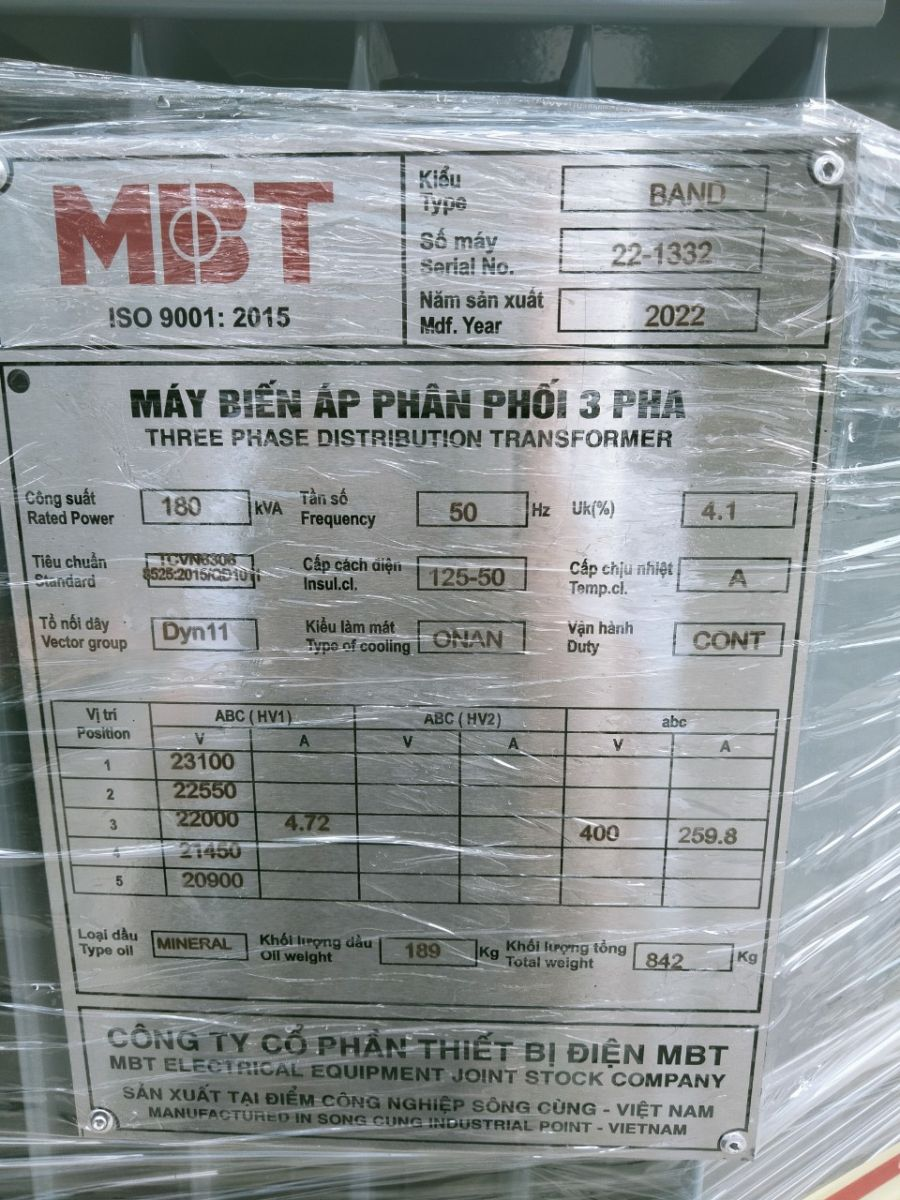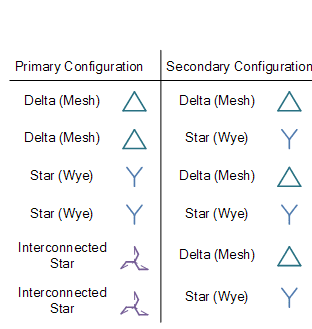Electrical transformers are basic equipment in the power grid. When an electrical device fails, it may be from a problem of the transformer. You must be sure that you have sized the transformer so as not to ruin that particular piece of equipment. Sizing a transformer is an easy task once you understand the nomenclature or language used. There is no hidden code on the transformer, just the abbreviations.

1. Transformer Nameplate

2. Parameters considered during the sizing of transformers

KVA Rating

Operational frequency

Winding connections

Other factors

3. Four steps to size a transformer

## 1. Transformer NameplateMBT Transformer nameplate

Transformer selection and sizing is the process of determining basic transformer parameters such as kVA, primary and secondary voltages and operating frequency. In addition to this winding material, winding connection, cooling method, power factor, mounting arrangement, type and performance are also considered in the selection process.

## 2. Parameters considered during the sizing of transformers

KVA Rating

During the selection and sizing of transformers, caculation of kVA in transformer is crucial. kVA is determined by the maximum apparent power which a transformer can handle.It relies on load current, voltage and power factors. It can be calculated using the following formula:

For single-phase transformers:

For three phase transformer,

Operational frequency

All transformers are designed for optimal flux linkages and the lowest level of heating and power loss at a given frequency. An induced potential difference is generated by the alternating magnetic field. At frequencies higher than the nominal frequency, excessive core loss is caused by lower efficiency in flux links. At frequencies lower than rated, winding loss is excessive due to reduced flux linkage.

Transformers are basically inductors:

• Increasing frequency increases impedance and reduces current (less flux).

• Lowering frequency lowers impedance and increases winding current (more heat).

Winding connections

Winding connections are not a problem for single-phase transformers. But with three phase transformers, the choice of winding connection is very important. Various primary and secondary winding configurations are shown below:Transformer winding connection

Other factor:

In addition to the above factors, many other factors such as transformer type (whether dry or oil-immersed), power factor, voltage regulation, operating temperature, installation arrangement, cooling arrangement and the impulse withstand capacity of the transformer is also taken into account in the selection process.

## 3. Four steps to size a transformer

Step 1

The first step is you need to understand the language used in the transformer. Most transformers types have the same terminology used to size the transformer. They are easy to understand since they use abbreviations: Voltage is V and amperage is A. The letter K represents Kilo which is equal to 1000. If a transformer has rated power at 120VA it means that it can handle 120 volts at 1-ampere or 1 amp of current. VA stands for Volt-Ampere, a symbol for power. A transformer rated at 1.2 KVA is another way of saying 1200 VA or 120 volts at 10 amps of current.

Step 2

The second step is you should calculate the load which places on the transformer. All electrical appliances have the rated wattage on the nameplate. They are always described as voltage and load amps. Load amps are calculated after the device is up and running. All electrical equipment has inrush currents and must be taken into account when sizing transformers. When calculating loads, always multiply the voltage by the load current and multiply the starting factor by 125 percent for the load.

## Step 3

Calculate an example as follows. A 120 volt motor has a load current of 5 amps. Multiplying 120 volts by 5 amps, which is equivalent to 600VA now gives 125 percent of the starting factor. Taking 600 times 1.25 equates to 720VA, and most transformers are sized by a factor of 25VA or 50VA. The required transformer will be a 750VA or .75KVA transformer. Always calculate VA power a little higher, never lower.

## Step 4

Size the transformer for any voltage or amperage. You can plug any voltage or current into your transformer's VA rating. The rating on the nameplate will have a maximum voltage rating for the transformer, usually 600 volts. If you want to operate a transformer at 24 volts and it is rated for 120VA, then divide 120 VA by 24 volts. The allowable amperage of the transformer will be 5 amps.

.(+84) 0913.006.538
Skype, Telegram, Wechat ...